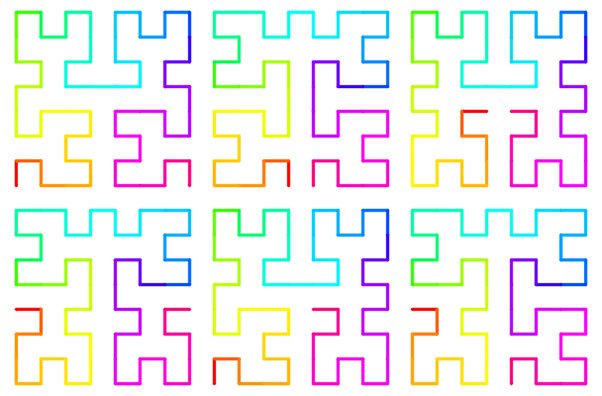## hilbert_2d

Functions for mapping between 1D and 2D space using the Hilbert curve, and its approximations

### 2 stable releases

 1.1.0 Jan 6, 2023 Sep 1, 2020

#96 in Math

Used in 6 crates (5 directly)

MIT/Apache

105KB
555 lines

# Hilbert 2D

Rust functions for mapping between 1D and 2D space using the Hilbert curve, and its approximations.## Usage

Add this to your `Cargo.toml`:

``````[dependencies]
hilbert_2d = "1.1.0"
``````

When working with images and matrices, use the `h2xy_discrete` and `xy2h_discrete` functions:

``````use hilbert_2d::{h2xy_discrete, xy2h_discrete, Variant};

let (x, y) = h2xy_discrete(7, 2, Variant::Hilbert); // (1, 2)
let h = xy2h_discrete(2, 1, 2, Variant::Hilbert); // 13
``````

When performing real-valued calculations, use the continuous functions instead:

``````use hilbert_2d::{h2xy_continuous_f64, Variant};

// Approaches the bottom-left corner
let (x1, y1) = h2xy_continuous_f64(0.0, Variant::Hilbert);
// Approaches the bottom-right corner
let (x2, y2) = h2xy_continuous_f64(1.0, Variant::Hilbert);
``````

Some of the pattern variants of the Hilbert curve have also been implemented:

``````use hilbert_2d::{h2xy_continuous_f64, Variant};

// In the Liu L1 variant, both ends of the curve approach the center of the square
let (x1, y1) = h2xy_continuous_f64(0.0, Variant::Liu1); // (~0.5, ~0.5)
let (x2, y2) = h2xy_continuous_f64(1.0, Variant::Liu1); // (~0.5, ~0.5)
``````